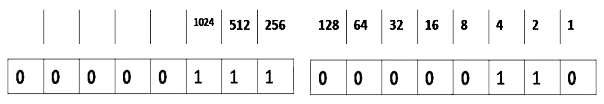# Technical Questions asked in ITC

1. What will be the output of the program ?
#include
int main()
{
union a
{
int i;
char ch;
}
union a u;
u.ch=6;
u.ch=7;
printf("%d\n", u.i);
return 0;
}

The system will allocate 2 bytes to the array1. Write a program to find factorial of a number.
int main()
{
int x,i,fac;
fac=1;
printf("input the number whose factorial has to be found");
scanf("%d", &x);
for(i=x; i>0,i--)
{
fac= fac*i;
}
u.i is calculated as
(6)(7) in binary form which is equal to 1798.
2+4+256+512+1024=1798
4. None of the above
3. Which of the following is a branch of polymorphism?
2. Inheritance
3. Encapsulation
4. None of these